# Including uncertainty in growth predictions in biogrowth

library(biogrowth)
library(tidyverse)

In most situations, growth predictions are surrounded by different sources of uncertainty and variability. For this reason, discrete growth predictions (i.e. growth curves) can be, in some cases, misleading. Consequently, biogrowth includes several functions to calculate growth predictions accounting for parameter uncertainty. Namely, it can account for the uncertainty of parameter estimates of primary growth models defined manually using predict_growth_uncertainty(). Also, it can include the uncertainty of a model fitted using a Monte Carlo algorithm with the predictMCMC() method.

## Growth predictions with uncertainty using predict_growth_uncertainty()

The function predict_growth_uncertainty() allows the definition of the distribution of the parameters of the primary growth model. Then, it includes this uncertainty in the model predictions through Monte Carlo simulations. It has 6 arguments:

• model_name defines the primary growth model,
• times defines the time points where to make the calculations,
• n_sims defines the number of Monte Carlo simulations,
• pars defines the distribution of the model parameters,
• corr_matrix correlation matrix between the model parameters. By default, this argument is set to an identity matrix (i.e. no correlation between parameters).
• check states whether to do validity checks of the model parameters (TRUE by default).

The calculations are done by taking a sample of size n_sims of the model parameters according to a multivariate normal distribution. For simulation, the population growth is predicted and the quantiles of the predicted population size is used as an estimate of the credible interval.

For this example, we will use the modified Gompertz model

my_model <- "modGompertz"

The pars argument defines the distribution of the model parameters. It must be a tibble with 4 columns (par, mean, sd and scale) and as many rows as model parameters. Then, for the modified Gompertz model, we will need 4 rows. The column par defines the parameter that is defined on each row. It must be a parameter identifier according to primary_model_data(). This function considers that each model parameter follows a marginal normal distribution with the mean defined in the mean column and the standard deviation defined in sd. This distribution can be defined in log-scale (by setting the value in scale to “log”), square-root scale (“sqrt”) or in the original scale (“original”). Note that, in order to omit the variability/uncertainty of any model parameter, one just has to set its corresponding standard error to zero.

pars <- tribble(
~par, ~mean, ~sd, ~scale,
"logN0", 0, .2, "original",
"mu", 2, .3, "sqrt",
"lambda", .5, .1, "log",
"C", 6, .5, "original"
)

For the time points, we will take 100 points uniformly distributed between 0 and 15:

my_times <- seq(0, 15, length = 100)

For the example, we will set the number of simulations to 1000. Nevertheless, it is advisable to repeat the calculations for various number of simulations to ensure convergence.

n_sims <- 1000

Once the arguments have been defined, we can call the predict_growth_uncertainty() function.

unc_growth <- predict_growth_uncertainty(my_model, my_times, n_sims, pars)

Before doing any calculations, predict_growth_uncertainty() makes several consistency checks of the model parameters (this can be turned off by passing check=FALSE). It returns an instance of GrowthUncertainty with the results of the simulation. It implements several S3 methods to facilitate the interpretation of the model simulations. The print method provides an overview of the simulation setting.

print(unc_growth)
#> Growth prediction based on primary models with uncertainty
#>
#> Primary model: modGompertz
#>
#> Mean values of the model parameters:
#>  0.0 2.0 0.5 6.0
#>
#> Variance-covariance matrix:
#>      [,1] [,2] [,3] [,4]
#> [1,] 0.04 0.00 0.00 0.00
#> [2,] 0.00 0.09 0.00 0.00
#> [3,] 0.00 0.00 0.01 0.00
#> [4,] 0.00 0.00 0.00 0.25

It also implements an S3 method for plot that can be used to visualize the credible intervals

plot(unc_growth)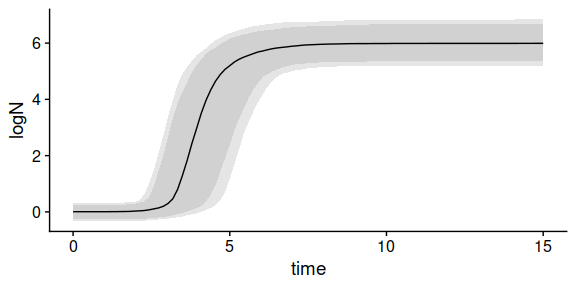In this plot, the solid line represents the mean of the simulations. Then, the two shaded areas represent, respectively, the space between the 10th and 90th, and the 5th and 95th quantiles.

The plot method includes additional arguments to edit the aesthetics of the plot.

plot(unc_growth, ribbon80_fill = "purple", ribbon90_fill = "pink", alpha80 = .8)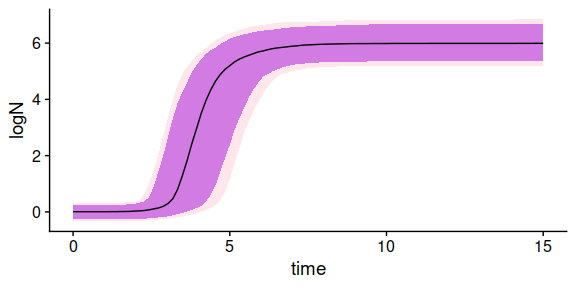Note that GrowthUncertainty is a subclass of list, making it easy to access several results of the simulation. Namely, it includes the following items:

• sample: sample of model parameters used for the simulations
• simulations: results of the individual simulations
• quantiles: quantiles of the population size predicted in the simulations
• model: model used for the simulations
• mus: expected values of the model parameters used for the simulations.
• sigma: variance-covariance matrix used for the simulations

By default, the function considers that there is no correlation between the model parameters. This can be varied by defining a correlation matrix. Note that the rows and columns of this matrix are defined in the the same order as in pars, and the correlation is defined in the scale of pars. For instance, we can define a correlation of -0.7 between the square root of $$\mu$$ and the logarithm of $$\lambda$$:

my_cor <- matrix(c(1,   0,    0, 0,
0,   1, -0.7, 0,
0, -0.7,   1, 0,
0,    0,   0, 1),
nrow = 4)

Then, we can include it in the call to the function

unc_growth2 <- predict_growth_uncertainty(my_model, my_times, n_sims, pars, my_cor)

plot(unc_growth2)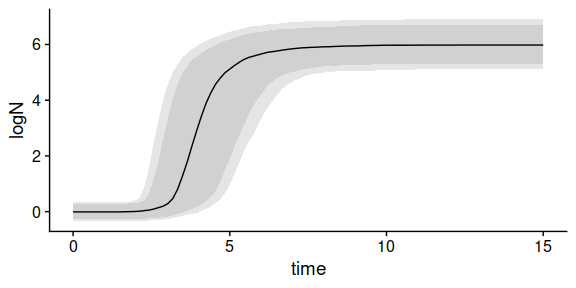## Accounting for the uncertainty of a fitted model using predictMCMC()

An alternative approach to account for uncertainty in model predictions is to use the distribution of the model parameters estimated from experimental data. In biogrowth, this can be done using the predictMCMC() S3 method of GrowthFit or GlobalGrowthFit. Note that this function is only available for models fitted using an Adaptive Monte Carlo algorithm (i.e., algorithm="MCMC"). This function takes 5 arguments:

• model the instance of GrowthFit or GlobalGrowthFit to use for the calculations
• times a numeric vector stating the time points where the solutions are calculated
• env_conditions a tibble (or data.frame) describing the values of the environmental conditions
• niter the number of Monte Carlo simulations
• newpars a named list defining values for some model parameters

First, we need a model fitted using fit_growth(). In this example, we will use a model fitted using approach="global". Nonetheless, the calculations of the predictions are exactly the same for a model fitted using approach="single". Note that, in this case, the model is fitted using an Adaptive Monte Carlo algorithm by setting algorithm="MCMC". This uses FME::modMCMC() instead of FME::modFit() for model fitting. We encourage the reader to read in detail the documentation of this function and references therein, as the interpretation of this fitting algorithm has several differences with respect to regression.

The following code chunk fits the growth model using a global approach. For a detailed description of the fit_growth() function, the reader is referred to the vignette dedicated to model fitting.

## We will use the data included in the package

data("multiple_counts")
data("multiple_conditions")

## We need to assign a model equation for each environmental factor

sec_models <- list(temperature = "CPM", pH = "CPM")

## Any model parameter (of the primary or secondary models) can be fixed

known_pars <- list(Nmax = 1e8, N0 = 1e0, Q0 = 1e-3,
temperature_n = 2, temperature_xmin = 20,
temperature_xmax = 35,
pH_n = 2, pH_xmin = 5.5, pH_xmax = 7.5, pH_xopt = 6.5)

## The rest, need initial guesses

my_start <- list(mu_opt = .8, temperature_xopt = 30)

set.seed(12421)
global_MCMC <- fit_growth(multiple_counts,
sec_models,
my_start,
known_pars,
environment = "dynamic",
algorithm = "MCMC",
approach = "global",
env_conditions = multiple_conditions,
niter = 100,
lower = c(.2, 29),  # lower limits of the model parameters
upper = c(.8, 34)  # upper limits of the model parameters
)
#> number of accepted runs: 13 out of 100 (13%)

Note that the number of iterations used in the model is too low for convergence of the fitting algorithm. This can be visualized by inspecting the trace plot of the Markov chain:

plot(global_MCMC$fit_results)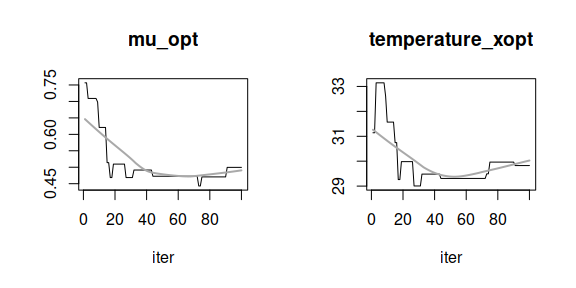Therefore, this model should only be considered as an illustration of the functions included in the package, not as a “serious” model. For additional details on how to evaluate the convergence of this fitting algorithm, the reader is referred to the documentation of FME and references therein. Once the model has been fitted, we can define the settings for the simulation. The predictMCMC() method requires the definition of the variation of the environmental conditions during the simulation. This is defined as a tibble (or data.frame) with the same conventions as for fit_growth(). Note that this argument must defined the same environmental factors as the ones considered in the original model. In this example, we will describe a constant environmental profile where temperature=30 and pH=7. Note that this is just an example, and dynamic profiles can also be calculated. my_conditions <- tibble(time = c(0, 40), temperature = c(30, 30), pH = c(7, 7) ) Then, we need to define the time points where the solution is calculated. We will use a uniformly distributed vector of length 50 between 0 and 40 my_times <- seq(0, 40, length = 50) The last argument we need to define is the number of Monte Carlo simulations to use for the calculations. The calculations are performed by taking a sample of size niter from the Markov chain of the model parameters. Then, for each parameter vector, the functions calculates the corresponding growth curve at each time point defined in times. For this example we will use 50 Monte Carlo simulations. This value is extremely low, and should only be used as an illustration of the implementation of the function. niter <- 50 Once every argument has been defined, we can call the predictMCMC() method set.seed(124) uncertain_prediction <- predictMCMC(global_MCMC, my_times, my_conditions, niter = niter ) The function returns an instance of MCMCgrowth with the results of the simulation. It includes several S3 methods to facilitate the interpretation of the results. The print method provides an overview of the simulation settings. print(uncertain_prediction) #> Growth prediction under dynamic conditions with parameter uncertainty #> #> Environmental factors included: time, temperature, pH #> #> Simulations based on the following model: #> #> Growth model fitted to data following a global approach conditions using MCMC #> #> Number of experiments: 2 #> #> Environmental factors included: temperature, pH #> #> Secondary model for temperature: CPM #> Secondary model for pH: CPM #> #> Parameter estimates: #> mu_opt temperature_xopt #> 0.5095069 29.9829208 #> #> Fixed parameters: #> Nmax N0 Q0 temperature_n #> 1.0e+08 1.0e+00 1.0e-03 2.0e+00 #> temperature_xmin temperature_xmax pH_n pH_xmin #> 2.0e+01 3.5e+01 2.0e+00 5.5e+00 #> pH_xmax pH_xopt #> 7.5e+00 6.5e+00 #> Parameter mu defined in log-10 scale #> Population size defined in log-10 scale The plot() method illustrates the distribution of the population size during the experiment. plot(uncertain_prediction)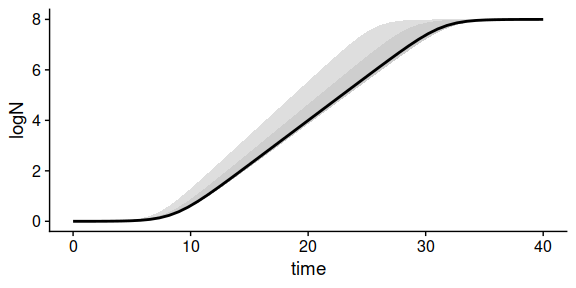The solid line illustrates the median of the simulations for each time point. Then, the ribbons show the space between the 10th and 90th, and 5th and 95th percentiles of the simulations for each time point. MCMCgrowth is defined as a subclass of list, so it is easy to access several aspects of the simulations. Namely, it has 5 entries: • sample a tibble with the sample of model parameters used for the simulations. • simulations a tibble with the results of every individual simulation used. • quantiles a tibble providing the calculated quantiles (5th, 10th, 50th, 90th, 95th) of the population size for each time point. • model the instance of FitDynamicGrowthMCMC used for the predictions. • env_conditions a tibble with the environmental conditions of the simulations. As already mentioned, the newpars argument can be used to define new values of the model parameters. As an illustration, we can fix mu_opt=0.5. uncertain_prediction2 <- predictMCMC(global_MCMC, my_times, my_conditions, niter = 5, newpars = list(mu_opt = 0.5) ) Inspecting the sample entry of the outcome shows that the value of mu_opt is fixed to 0.5 for these simulations, whereas temperature_xopt varies according to the fitted model. uncertain_prediction2$sample
#> # A tibble: 5 × 3
#>   mu_opt temperature_xopt  iter
#>    <dbl>            <dbl> <int>
#> 1    0.5             29.3     1
#> 2    0.5             29.5     2
#> 3    0.5             29.5     3
#> 4    0.5             29.0     4
#> 5    0.5             29.3     5

## Accounting for uncertainty when calculating the time to reach a population size

The biogrowth package includes the time_to_size() function to estimate the elapsed time required to reach a given population size for growth models. By default, this function calculates a discrete value for the elapsed time. Nevertheless, this can be modified passing type=distribution. In this case, the function takes two arguments:

• model an instance of GrowthUncertainty or MCMCgrowth
• size the target population size (in log units)

The distribution of the time to reach the given is estimated by linear interpolation for each of the growth curves included in model. Therefore, its precision is strongly dependent on the number of simulations (i.e. the number of growth curves) and the density of the time points around the solution. For that reason, considering the low number of iterations and time points included in the simulations, the results presented here should only be considered as an illustration of the functions.

unc_distrib <- time_to_size(type = "distribution", unc_growth, 3)

The function returns an instance of TimeDistribution with the results of the calculation. It includes several S3 methods to facilitate the interpretation of the results. The print method provides an overall view of the results

print(unc_distrib)
#> Distribution of the time required to reach a target population size
#>
#> # A tibble: 1 × 5
#>   m_time sd_time med_time   q10   q90
#>    <dbl>   <dbl>    <dbl> <dbl> <dbl>
#> 1   3.98   0.813     3.94  3.03  5.15

The summary method provides a table with several statistical indexes of the distribution of the time.

summary(unc_distrib)
#> # A tibble: 1 × 5
#>   m_time sd_time med_time   q10   q90
#>    <dbl>   <dbl>    <dbl> <dbl> <dbl>
#> 1   3.98   0.813     3.94  3.03  5.15

The plot method shows an histogram of the distribution. In this plot, the median of the simulations is shown as a dashed, red line. The 10th and 90th percentiles are shown as grey, dashed lines.

plot(unc_distrib)
#> stat_bin() using bins = 30. Pick better value with binwidth.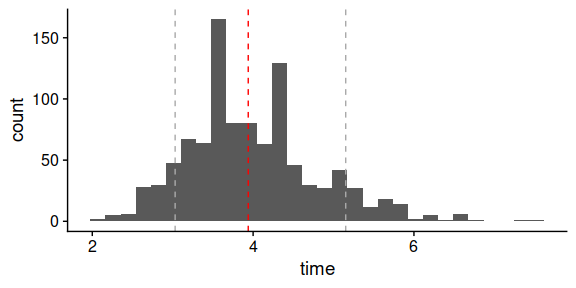When interpreting the results of the calculation is important to consider how NAs are considered by the function. It is possible that, for some simulations, the target population size is not included in the growth curve. In that case, that particular calculation would results in a time of NA. This value is then omitted when making the calculations (medians, quantiles, histograms…). This can be relevant for population size in the edge of the simulations (e.g. close to logN0 or logNmax) and can introduce a bias in the results.